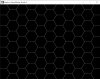# Hex Grid Code Refactoring and Maths Help

#### tagwolf

##### Member
Hello, I am trying to refactor and cleanup my code to plug in a reusable and resizeable algorithm (including padding between hexcells). Unfortunately, I don't understand the maths well enough to get it done, but I have a static product that is working right now. hexcell is pretty much where I want it outside of the top-left x,y not mapping to where I want it. It'd also be nice to be able to easily flip the hex orientation. Any help would be much appreciated. Especially why my spacingh variable has to be -9....

The main part I need help with is the formula for spacing hexagons of any size/padding every other line and how that translates to gml.obj_hexgrid - create
So many hardcoded valuesCode:
``````gridw = 8;
gridh = 16;
spacingw = 64;
spacingh = -9;

for (i = 0; i < gridw; i ++)
{
for (j = 0; j < gridh; j ++)
{
if (j % 2 == 0)
{
instance_create_layer((x + spacingw) * i, (y + spacingh) * j, layer, obj_hexcell);
}
else
{
instance_create_layer((x + spacingw) * i + 96, (y + spacingh) * j, layer, obj_hexcell);
}
}
}``````
obj_hexcell - create
Code:
``````sides = 6;
sidelength = 64;
angle = 360/sides;

newangle = 0;
newx = x;
newy = y;``````
obj_hexcell - draw
Code:
``````draw_primitive_begin(pr_linestrip)
repeat(sides)
{
newangle += angle;
if newangle >= 360 {newangle = 0;}
oldx = newx;
oldy = newy;
newx = oldx - lengthdir_x(sidelength, newangle);
newy = oldy + lengthdir_y(sidelength, newangle);
draw_vertex(oldx,oldy);
}
draw_primitive_end();``````

Last edited:

#### van0014

##### Member
A negative value suggests a flaw in the algorithm. It's important to eliminate this constant and calculate the values instead. Symmetrical hexagons share properties with circles, such as diameter. If the algorithm was perfected, you could set the radius to 64 to achieve the image in your screenshot; That shows hexagons with a width / diameter of 128 pixels, not the 64 pixels defined.

Your code easily creates hexagons, though they use different variables from the hexgrid object. These need to be linked.
The hexagons are offset by more than half their diameter. You would need to calculate the spacing of arbitrary hexagon sizes in order to tessellate them. That means finding a ratio that results in proper tessellation of any sized hexagon.

•tagwolf

#### TailBit

##### Member
The height from the center to the top is sin(60 degree) * 64 or sqrt(3)/2*64 .. if you then take away the radius of the circle:
= 55.42 - 64 = -8.57
So the -9 is not too far of for drawing it perfectly in line, as that is just the top of the circle shape that is cut off

the left and right side is both 1/4th of the total width 128 .. and you start drawing it from the bottom left; at 3/4 width .. so:
spacingw = 128/4*3;

I would recommend you to have a look at this goldmine for hexagon knowledge: https://www.redblobgames.com/grids/hexagons/

I've been using it with good results so far, drawing odd-r hexagons for my inventory, but drawing images instead of lines makes it hard to avoid gaps ..

Last edited:
•tagwolf